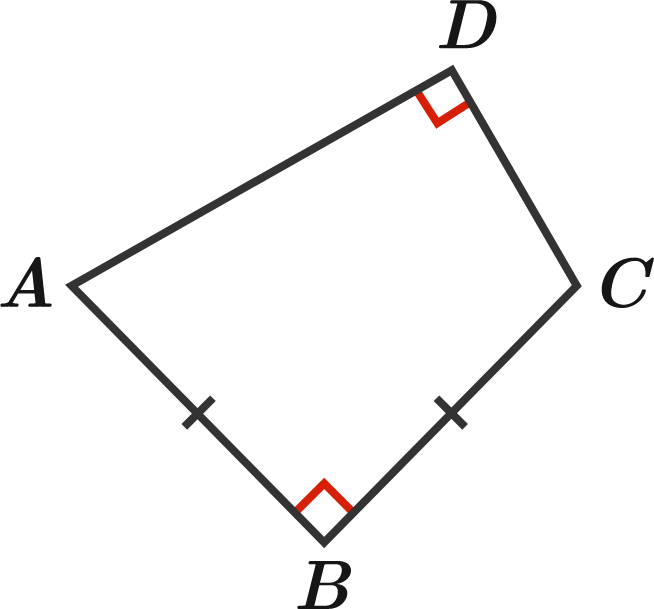# The area is constant?

Geometry Level 1Quadrilateral $ABCD$ has the following properties:

• Opposite angles $B$ and $D$ are each $90^\circ.$
• $AB$ and $BC$ have the same length.
• $AD+DC=1.$

What can be stated about other quadrilaterals that have the same properties as $ABCD?$

×